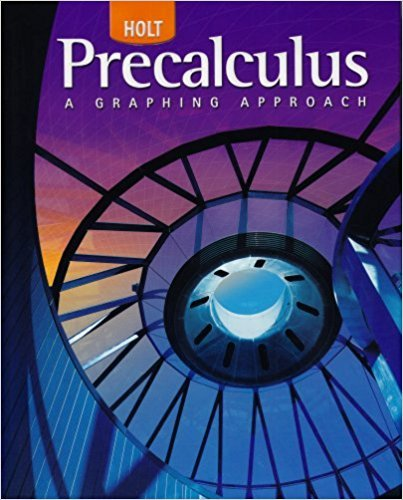×

×

Textbooks / Calculus / Precalculus 1

# Precalculus 1st Edition - Solutions by Chapter## Full solutions for Precalculus | 1st Edition

ISBN: 9780030416477Precalculus | 1st Edition - Solutions by Chapter

Solutions by Chapter
4 5 0 327 Reviews
##### ISBN: 9780030416477

Since problems from 99 chapters in Precalculus have been answered, more than 86711 students have viewed full step-by-step answer. This expansive textbook survival guide covers the following chapters: 99. This textbook survival guide was created for the textbook: Precalculus, edition: 1. The full step-by-step solution to problem in Precalculus were answered by , our top Calculus solution expert on 03/16/18, 04:19PM. Precalculus was written by and is associated to the ISBN: 9780030416477.

Key Calculus Terms and definitions covered in this textbook

If u < v , then u + w < v + w

• Blind experiment

An experiment in which subjects do not know if they have been given an active treatment or a placebo

• Common difference

See Arithmetic sequence.

• Elimination method

A method of solving a system of linear equations

• Half-life

The amount of time required for half of a radioactive substance to decay.

• Inductive step

See Mathematical induction.

• Inverse cotangent function

The function y = cot-1 x

• Linear regression line

The line for which the sum of the squares of the residuals is the smallest possible

• Magnitude of a vector

The magnitude of <a, b> is 2a2 + b2. The magnitude of <a, b, c> is 2a2 + b2 + c2

• Negative linear correlation

See Linear correlation.

• Objective function

See Linear programming problem.

• Open interval

An interval that does not include its endpoints.

The formula x = -b 2b2 - 4ac2a used to solve ax 2 + bx + c = 0.

A procedure for fitting a quadratic function to a set of data.

• Real number line

A horizontal line that represents the set of real numbers.

• Regression model

An equation found by regression and which can be used to predict unknown values.

• Secant line of ƒ

A line joining two points of the graph of ƒ.

• Standard deviation

A measure of how a data set is spread

• Standard form of a polynomial function

ƒ(x) = an x n + an-1x n-1 + Á + a1x + a0

• Zero factor property

If ab = 0 , then either a = 0 or b = 0.